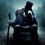# Question from Elementary Number Theory by David M. Burton

Hello

I was solving the $3rd$ question from the $1st$ chapter from the book. I squeezed my brain but I'm unable to figure out how to start proving this.

Any help is welcome.

The Problem:

Use the Second Principle of Finite Induction to establish that $a^n-1=(a-1)(a^{n-1}+a^{n-2}+a^{n-3}+...+a+1)$ for all $n>=1$. [Hint: $a^{n+1}-1=(a+1)(a^n-1)-a(a^{n-1}-1))$ ]

(P.S. I don't need complete proof, I just want to know how to start. If I'm too noob to figure out things after that, I'll ask for complete solution if some would kindly provide :) )Note by Kou\$htav Chakrabarty
7 years, 1 month ago

This discussion board is a place to discuss our Daily Challenges and the math and science related to those challenges. Explanations are more than just a solution — they should explain the steps and thinking strategies that you used to obtain the solution. Comments should further the discussion of math and science.

When posting on Brilliant:

• Use the emojis to react to an explanation, whether you're congratulating a job well done , or just really confused .
• Ask specific questions about the challenge or the steps in somebody's explanation. Well-posed questions can add a lot to the discussion, but posting "I don't understand!" doesn't help anyone.
• Try to contribute something new to the discussion, whether it is an extension, generalization or other idea related to the challenge.
• Stay on topic — we're all here to learn more about math and science, not to hear about your favorite get-rich-quick scheme or current world events.

MarkdownAppears as
*italics* or _italics_ italics
**bold** or __bold__ bold
- bulleted- list
• bulleted
• list
1. numbered2. list
1. numbered
2. list
Note: you must add a full line of space before and after lists for them to show up correctly
paragraph 1paragraph 2

paragraph 1

paragraph 2

[example link](https://brilliant.org)example link
> This is a quote
This is a quote
    # I indented these lines
# 4 spaces, and now they show
# up as a code block.

print "hello world"
# I indented these lines
# 4 spaces, and now they show
# up as a code block.

print "hello world"
MathAppears as
Remember to wrap math in $$ ... $$ or $ ... $ to ensure proper formatting.
2 \times 3 $2 \times 3$
2^{34} $2^{34}$
a_{i-1} $a_{i-1}$
\frac{2}{3} $\frac{2}{3}$
\sqrt{2} $\sqrt{2}$
\sum_{i=1}^3 $\sum_{i=1}^3$
\sin \theta $\sin \theta$
\boxed{123} $\boxed{123}$

## Comments

Sort by:

Top Newest

After proving the base cases for $n=1,2$, plug in the expansion of $a^{n}-1$ and $a^{n-1}-1$ (this is valid due to the induction assumption) in the formula given in the hint to get the result for $a^{n+1}-1$.

- 7 years, 1 month ago

Log in to reply

That seems like a pretty ridiculous hint! If I were going to use induction I'd use $a^{n+1}-1 = (a^n-1) + a^n(a-1)$ and then substitute the expansion in using the inductive hypothesis. Much cleaner.

- 7 years, 1 month ago

Log in to reply

Hi Patrick On a different matter can you prove for me that if the sum of two perfect squares is a perfect square then their difference can not be a perfect square Enjoy reading your solutions Des O Carroll

- 7 years, 1 month ago

Log in to reply

I'm not sure you're going to like this answer, Des! But here goes. Suppose $a^2+b^2=c^2$ and $a^2-b^2 = d^2$. This describes a curve in projective $3$-space (cut out by the intersection of two planes).

Set $y = 2b$, $x = 2(a-d)$, $z = 2a-c-d$ for all points on the curve except $(1,0,1,1).$ If $(a,b,c,d) = (1,0,1,1),$ set $(x,y,z) = (0,1,0).$ Then if I did the algebra right, $x,y,z$ satisfy the equation $y^2z= x(x-z)(x-2z).$ You may recognize this as an elliptic curve, and in fact this map gives an isomorphism between your original curve and the elliptic curve. Standard elliptic curve methods show that this curve has rank 0 and exactly four torsion points: $(0,1,0)$ plus the three points $(a,0,1)$ where $a = 0,1,2$. These points correspond to the points $(1,0,\pm 1, \pm 1)$ on the original curve. So the only rational solutions to your original equations are the "trivial" ones where $b = 0$.

This is kind of a punt because the "standard elliptic curve methods" are pretty deep. It's not hard to find the torsion subgroup, but showing that the rank is 0 is in general pretty tricky. I actually used a computer algebra system to verify this, but I'm sure there is a down-to-earth way to do it as well for this specific example.

Sorry if I did the algebra wrong! I am sure that the original curve and my elliptic curve are isomorphic (computer algebra system again!), but I haven't checked for sure that my isomorphism is correct.

- 7 years, 1 month ago

Log in to reply

Many thanks Patrick Im afraid that much of the solution is beyond me but it confirms that this is quite a difficult problem not accessible to an easy solution Anyway it was so kind of you to get back to me so quickly and thanks again Des O Carroll

- 7 years, 1 month ago

Log in to reply

×

Problem Loading...

Note Loading...

Set Loading...